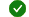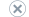Applies to:Visual StudioVisual Studio for MacVisual Studio Code

Item Value
RuleId CA2224
Category Microsoft.Usage
Breaking change Non-breaking

## Cause

A public type implements the equality operator, but does not override System.Object.Equals.

## Rule description

The equality operator is intended to be a syntactically convenient way to access the functionality of the Equals method. If you implement the equality operator, its logic must be identical to that of Equals.

The C# compiler issues a warning if your code violates this rule.

## How to fix violations

To fix a violation of this rule, you should either remove the implementation of the equality operator, or override Equals and have the two methods return the same values. If the equality operator does not introduce inconsistent behavior, you can fix the violation by providing an implementation of Equals that calls the Equals method in the base class.

## When to suppress warnings

It is safe to suppress a warning from this rule if the equality operator returns the same value as the inherited implementation of Equals. The examples in this article include a type that could safely suppress a warning from this rule.

## Examples of Inconsistent Equality Definitions

The following example shows a type with inconsistent definitions of equality. `BadPoint` changes the meaning of equality by providing a custom implementation of the equality operator, but does not override Equals so that it behaves identically.

``````using System;

namespace UsageLibrary
{
{
private int x,y, id;
private static int NextId;

{
NextId = -1;
}
{
this.x = x;
this.y = y;
}

public override string ToString()
{
return String.Format("([{0}] {1},{2})",id,x,y);
}

public int X {get {return x;}}

public int Y {get {return x;}}
public int Id {get {return id;}}

public override int GetHashCode()
{
return id;
}
// Violates rule: OverrideEqualsOnOverridingOperatorEquals.

// BadPoint redefines the equality operator to ignore the id value.
// This is different from how the inherited implementation of
// System.Object.Equals behaves for value types.
// It is not safe to exclude the violation for this type.
{
return ((p1.x == p2.x) && (p1.y == p2.y));
}
{
return !(p1 == p2);
}
}
}
``````

The following code tests the behavior of `BadPoint`.

``````using System;

namespace UsageLibrary
{
{
public static void Main()
{

Console.WriteLine("a =  {0} and b = {1} are equal? {2}", a, b, a.Equals(b)? "Yes":"No");
Console.WriteLine("a == b ? {0}", a == b ? "Yes":"No");
Console.WriteLine("a1 and a are equal? {0}", a1.Equals(a)? "Yes":"No");
Console.WriteLine("a1 == a ? {0}", a1 == a ? "Yes":"No");

// This test demonstrates the inconsistent behavior of == and Object.Equals.
Console.WriteLine("b and bcopy are equal ? {0}", bcopy.Equals(b)? "Yes":"No");
Console.WriteLine("b == bcopy ? {0}", b == bcopy ? "Yes":"No");
}
}
}
``````

This example produces the following output:

``````a =  ( 1,1) and b = ( 2,2) are equal? No
a == b ? No
a1 and a are equal? Yes
a1 == a ? Yes
b and bcopy are equal ? No
b == bcopy ? Yes
``````

The following example shows a type that technically violates this rule, but does not behave in an inconsistent manner.

``````using System;

namespace UsageLibrary
{
public struct GoodPoint
{
private int x,y;

public GoodPoint(int x, int y)
{
this.x = x;
this.y = y;
}

public override string ToString()
{
return String.Format("({0},{1})",x,y);
}

public int X {get {return x;}}

public int Y {get {return x;}}

// Violates rule: OverrideEqualsOnOverridingOperatorEquals,
// but does not change the meaning of equality;
//  the violation can be excluded.

public static bool operator== (GoodPoint px, GoodPoint py)
{
return px.Equals(py);
}

public static bool operator!= (GoodPoint px, GoodPoint py)
{
return !(px.Equals(py));
}
}
}
``````

The following code tests the behavior of `GoodPoint`.

``````using System;

namespace UsageLibrary
{
public class TestGoodPoint
{
public static void Main()
{
GoodPoint a = new GoodPoint(1,1);
GoodPoint b = new GoodPoint(2,2);
GoodPoint a1 = a;
GoodPoint bcopy = new GoodPoint(2,2);

Console.WriteLine("a =  {0} and b = {1} are equal? {2}", a, b, a.Equals(b)? "Yes":"No");
Console.WriteLine("a == b ? {0}", a == b ? "Yes":"No");
Console.WriteLine("a1 and a are equal? {0}", a1.Equals(a)? "Yes":"No");
Console.WriteLine("a1 == a ? {0}", a1 == a ? "Yes":"No");

// This test demonstrates the consistent behavior of == and Object.Equals.
Console.WriteLine("b and bcopy are equal ? {0}", bcopy.Equals(b)? "Yes":"No");
Console.WriteLine("b == bcopy ? {0}", b == bcopy ? "Yes":"No");
}
}
}
``````

This example produces the following output:

``````a =  (1,1) and b = (2,2) are equal? No
a == b ? No
a1 and a are equal? Yes
a1 == a ? Yes
b and bcopy are equal ? Yes
b == bcopy ? Yes
``````

## Class Example

The following example shows a class (reference type) that violates this rule.

``````using System;

namespace Samples
{
// Violates this rule
public class Point
{

public Point(int x, int y)
{
_X = x;
_Y = y;
}

public int X
{
get { return _X; }
}

public int Y
{
get { return _Y; }
}

public override int GetHashCode()
{
return _X ^ _Y;
}

public static bool operator ==(Point point1, Point point2)
{
if (point1 == null || point2 == null)
return false;

if (point1.GetType() != point2.GetType())
return false;

if (point1._X != point2._X)
return false;

return point1._Y == point2._Y;
}

public static bool operator !=(Point point1, Point point2)
{
return !(point1 == point2);
}
}
}
``````

The following example fixes the violation by overriding System.Object.Equals.

``````using System;

namespace Samples
{
public class Point
{

public Point(int x, int y)
{
_X = x;
_Y = y;
}

public int X
{
get { return _X; }
}

public int Y
{
get { return _Y; }
}

public override int GetHashCode()
{
return _X ^ _Y;
}

public override bool Equals(object obj)
{
if (obj == null)
return false;

if (GetType() != obj.GetType())
return false;

Point point = (Point)obj;

if (_X != point.X)
return false;

return _Y == point.Y;
}

public static bool operator ==(Point point1, Point point2)
{
return Object.Equals(point1, point2);
}

public static bool operator !=(Point point1, Point point2)
{
return !Object.Equals(point1, point2);
}
}
}
``````

## Structure Example

The following example shows a structure (value type) that violates this rule:

``````using System;

namespace Samples
{
// Violates this rule
public struct Point
{

public Point(int x, int y)
{
_X = x;
_Y = y;
}

public int X
{
get { return _X; }
}

public int Y
{
get { return _Y; }
}

public override int GetHashCode()
{
return _X ^ _Y;
}

public static bool operator ==(Point point1, Point point2)
{
if (point1._X != point2._X)
return false;

return point1._Y == point2._Y;
}

public static bool operator !=(Point point1, Point point2)
{
return !(point1 == point2);
}
}
}
``````

The following example fixes the violation by overriding System.ValueType.Equals.

``````using System;

namespace Samples
{
public struct Point : IEquatable<Point>
{

public Point(int x, int y)
{
_X = x;
_Y = y;
}

public int X
{
get { return _X; }
}

public int Y
{
get { return _Y; }
}

public override int GetHashCode()
{
return _X ^ _Y;
}

public override bool Equals(object obj)
{
if (!(obj is Point))
return false;

return Equals((Point)obj);
}

public bool Equals(Point other)
{
if (_X != other._X)
return false;

return _Y == other._Y;
}

public static bool operator ==(Point point1, Point point2)
{
return point1.Equals(point2);
}

public static bool operator !=(Point point1, Point point2)
{
return !point1.Equals(point2);
}
}
}
``````

CA1046: Do not overload operator equals on reference types

CA2225: Operator overloads have named alternates

CA2226: Operators should have symmetrical overloads

CA2218: Override GetHashCode on overriding Equals

CA2231: Overload operator equals on overriding ValueType.Equals